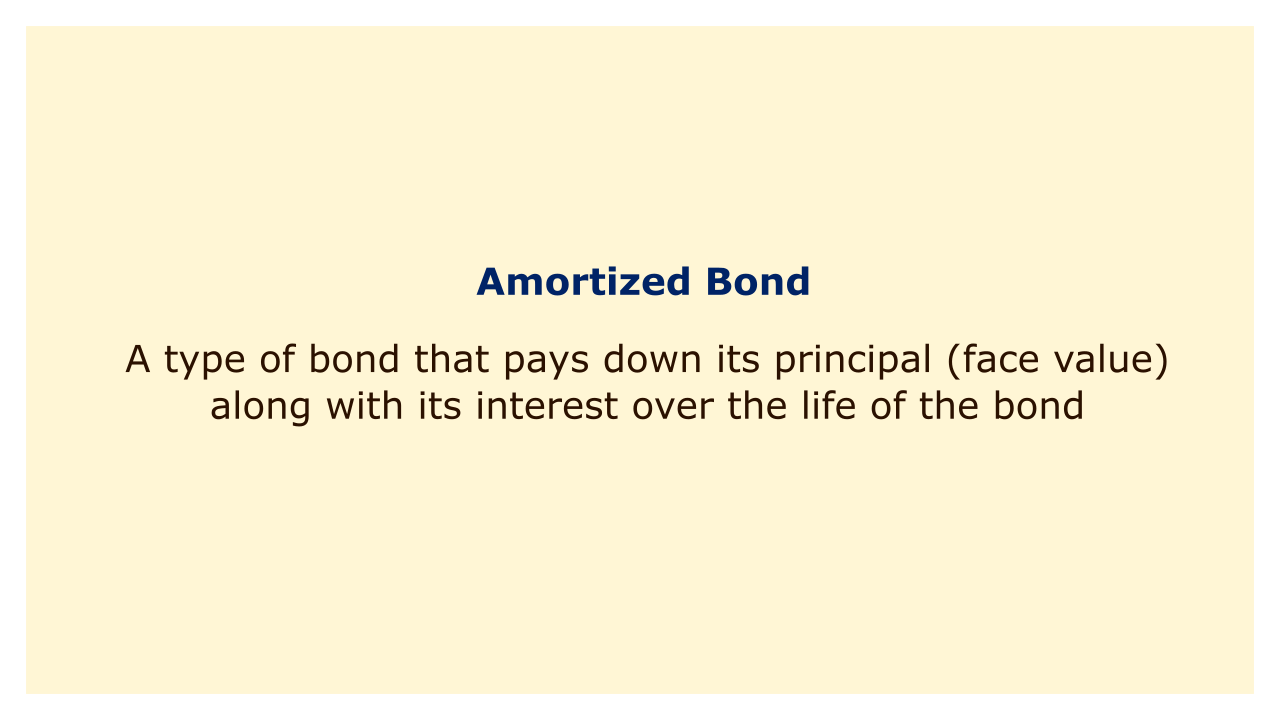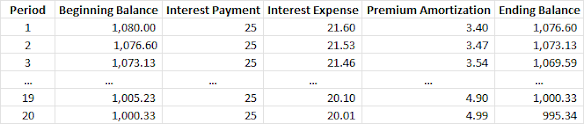# Amortized BondImage: Moneybestpal.com

### An amortized bond is a form of bond that pays off both its interest and principal over the course of its existence. As a result, each payment made by the issuer to the bondholder includes both interest and principal payments.

The principal portion of a bond increases over time until the bond is entirely repaid at maturity, whereas the interest portion is often fixed.

Unlike a bullet or balloon bond, which only pays interest throughout the bond's duration and repays the entire principal at maturity, an amortized bond recoups its entire principal over its entire life. A zero-coupon bond, which bears no interest and is sold at a substantial discount to its face value, is distinct from an amortized bond in other ways.

A fixed-rate home mortgage is an illustration of an amortized bond. Over the course of, say, 30 years, the monthly payment on such a loan remains consistent and includes both interest and principal. A zero loan balance at the conclusion of the term is achieved by calculating the payment in this way.

#### Benefits and drawbacks of amortized bonds

Amortized bonds have some advantages and disadvantages for both issuers and investors. Some of the benefits are:
• Amortized bonds minimize the issuer's credit risk because the principal is repaid gradually rather than all at once when the bond matures. As a result, the issuer faces less of a risk of default and refinancing.
• Due to their shorter length compared to non-amortized bonds with the same maturity and coupon rate, amortized bonds minimize the investor's interest rate risk. The duration of a bond expresses how responsive its price is to changes in interest rates. The bond's price is less erratic the shorter the duration.
• For the investor, amortized bonds offer a consistent flow of income and principal repayment that can be reinvested or put to other uses.

#### Some of the drawbacks are:

• Amortized bonds minimize the issuer's credit risk because the principal is repaid gradually rather than all at once when the bond matures. As a result, the issuer faces less of a risk of default and refinancing.
• Due to their shorter length compared to non-amortized bonds with the same maturity and coupon rate, amortized bonds minimize the investor's interest rate risk. The duration of a bond expresses how responsive its price is to changes in interest rates. The bond's price is less erratic the shorter the duration.
• For the investor, amortized bonds offer a consistent flow of income and principal repayment that can be reinvested or put to other uses.

#### Methods of amortization

Bond premiums and discounts can be amortized using either a straight line or an effective interest method. When a bond is issued, a bond premium happens when the price is higher than its face value, and a bond discount occurs when the price is lower. A cost or benefit that must be amortized during the bond's life for the issuer is represented by the difference between the issue price and face value.

The straight-line method is the simplest method, which allocates an equal amount of bond premium or discount to each period. The formula for calculating the bond premium or discount amortization using this method is:

Bond Premium or Discount Amortization = (Bond Issue Price - Bond Face Value) / Number of Periods

The effective-interest method is more accurate, but also more complicated, which allocates a varying amount of bond premium or discount to each period based on the effective interest rate. The formula for calculating the bond premium or discount amortization using this method is:

Bond Premium or Discount Amortization = Bond Carrying Value x (Effective Interest Rate - Coupon Rate)

The internal rate of return (IRR) that compares the issue price of the bond to the present value of all future cash flows from the bond is known as the effective interest rate. Bond carrying value is the bond's book value after deducting accrued amortization.

#### Example of amortizing a bond

Consider Company A issues a \$1,000 face value, 5% coupon, 10-year bond at a cost of \$1,080 while the market interest rate is 4%. The effective-interest approach is used to amortize the bond's premium, and interest is paid semi-annually.

The effective interest rate for this bond is 4% / 2 = 2% per period. The bond premium is \$1,080 - \$1,000 = \$80, which needs to be amortized over 20 periods (10 years x 2).

The amortization schedule for this bond is shown below:Image: Moneybestpal.com

As you can see, the amortization of the bond premium lowers the carrying value of the bond over time until it reaches the face value at maturity. Due to the bond's lower effective interest rate, the interest expense is always less than the monthly payout.
Tags